Courses

# Test: Time And Work- 2

## 20 Questions MCQ Test Quantitative Aptitude for GMAT | Test: Time And Work- 2

Description
This mock test of Test: Time And Work- 2 for UPSC helps you for every UPSC entrance exam. This contains 20 Multiple Choice Questions for UPSC Test: Time And Work- 2 (mcq) to study with solutions a complete question bank. The solved questions answers in this Test: Time And Work- 2 quiz give you a good mix of easy questions and tough questions. UPSC students definitely take this Test: Time And Work- 2 exercise for a better result in the exam. You can find other Test: Time And Work- 2 extra questions, long questions & short questions for UPSC on EduRev as well by searching above.
QUESTION: 1

### At a food processing and packaging plant, identical packets of confectionary items are prepared at a constant rate by a packaging machine. Each packet contains 3 chocolates, 4 candied nuts and 2 chewing gums. The production rate of chocolates, candied nuts and chewing gums at the plant is 750, 975 and 575 units per hour respectively. All the produced items are immediately sent to a common quality checking machine that can process 3000 units of confectionary items per hour. 20 percent each of chocolates, candied nuts and chewing gums fail the quality check. The remaining items are sent to the packaging plant without any holdup or delay. Which of the following can represent the number of packets of confectionary items prepared by the packaging machine in one hour? I. 195 II. 230 III. 280

Solution:

Given:

• Contents of 1 packet:
• Chocolates = 3
• Candied Nuts = 4
• Chewing Gums = 2
• The production rate (number of units produced per hour) is as under: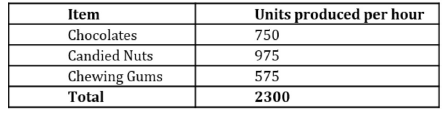• The maximum units that the quality checking machine can process in 1 hour = 3000
• Since the total number of items produced per hour is only 2300 (less than 3000), this means, there is no holdup/ delay at the quality checking machine. The machine is able to immediately process each produced item.
• 20% each of Chocolates, Candied Nuts and Chewing Gums fail the test This means, only 80% of the produced Chocolates, Candied Nuts and Chewing Gums go to the packaging plant.

To Find:  Can the number of packets prepared by the packaging machine in 1 hour be {195, 230, 280}?

Approach:

1. To answer the question, we first need to understand what are the constraints on the number of packets prepared by the packaging machine in 1 hour.
• Constraint 1 – The processing capacity of the packaging machine. For example, say the packaging machine is only able to prepare 100 packets per hour.
• Constraint 2 – The number of Chocolates, Candied Nuts and Chewing Gums available to the packaging machine per hour.
• For example, say the packaging machine has the capacity to prepare 10,000 packets per hour but only 30 chocolates come into the packaging machine in one hour. This means, the packaging machine can only produce 10 packets in one hour (since each packet must contain 3 chocolates). This will be the case if the number of Candied Nuts and Chewing Gums is not a limiting factor.
• Now assume a further scenario where only 30 chocolates, 8 candied nuts and 10 chewing gums come into the packaging machine per hour. In this case, the packaging machine can only produce 2 packets per hour (since each packet must contain 4 candied nuts). The number of candied nuts proved to a limiting factor in this scenario.
2. So, our approach will be to first find the number of Chocolates, Candied Nuts and Chewing Gums available to the packaging machine per hour (as inferred in the Given section, this number is equal to 80% of the respective production rates of these 3 items)
3. Next, we’ll find which of these 3 items acts as a limiting factor, and will find the number of packets that are produced per hour in the case of the limiting factor.

Working out: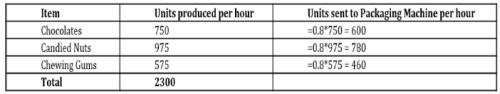• So, each hour the packaging machine gets 600 Chocolates, 780 Candied Nuts and 460 Chewing Gums.
• Once again, remember that each packet contains 3 Chocolates, 4 Candied Nuts and 2 Chewing Gums.
• So, the maximum number of packets that can be packaged in 1 hour =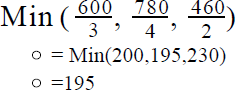• Note that Candied Nuts acted as a limiting factor in the number of packets produced per hour.
• So, given the current production (and quality check failing) rates of Chocolates, Candied Nuts and Chewing Gums, the maximum number of packets that can be produced per hour is 195.
• The production will be 195 packets per hour if the processing capacity of the packaging machine is not the limiting factor (that is, Constraint 1 doesn’t come into play, as in the example we considered above where the machine was even capable of packaging 10,000 packets per hour but was limited in its actual output by the number of Chocolates, Candied Nuts and Chewing Gums that were available to it per hour)
• However, if the processing capacity of the packaging machine is the limiting factor, then the actual number of packets produced will be less than 195.
• For example, say the packaging machine can only process 50 packets in 1 hour. Then, even though it is getting enough Chocolates, Candied Nuts and Chewing Gums to be able to pack 195 packets in one hour, it will only be able to pack 50 packets in 1 hour (resulting in a huge stockpiling of Chocolates, Candied Nuts and Chewing Gums at the site of the packaging machine!)
• Thus, the number of packets produced per hour will be less than or equal to 195.
• Out of the 3 given values, the only value possible is Value 1 (195)
Looking at the answer choices, we see that the correct answer is Option A
QUESTION: 2

### Operating at their respective constant rates, Photocopying machine B takes 6 minutes more than photocopying machine A to copy x pages. When machines A and B are operated simultaneously, 7x pages can be copied in 20 minutes. In how many minutes can machine A operating alone copy 2x pages?

Solution:

Given: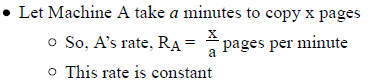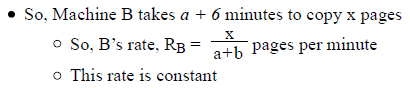• Time taken by A and B together to copy 7x pages) = 20 minutes

To Find: Time taken by A alone to copy 2x pages

Approach: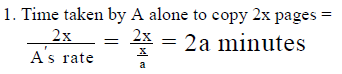2. We’re given the combined output of A and B and the time taken for this output. Using this data, we can find the combined rate of A and B.
Then, we’ll use the equation (Combined Rate of A and B) = (Rate of A) + (Rate of B) to get an equation in terms of a.

Working out:

• When A and B operate simultaneously, 7x pages take 20 minutes
• So, Combined Rate =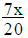pages per minute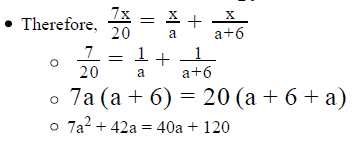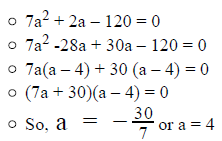• The negative root is rejected since time cannot be negative.
• So, a = 4 minutes
• Therefore, Required Time = 2a = 2*4 = 8 minutes

Looking at the answer choices, we see that the correct answer is Option B

QUESTION: 3

### Peter, Mark and John work in the marketing department of an e-learning firm. Peter can answer 25 emails in an hour, Mark can answer 40 percent more emails in an hour than Peter and John takes 1/6  lesser time to answer the same number of emails as Peter. By what percentage should Peter increase his speed so that the three employees can together answer 1/6 more emails than they currently do?

Solution:

Given:

• Number of emails answered by Peter in 1 hour = 25
• Number of emails answered by Mark in 1 hour = 25 + 40% of 25
• Time taken by John to answer 25 emails =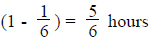To Find: Percentage by which Peter should increase his speed so that all 3 can answer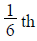more emails than they currently do?

Approach: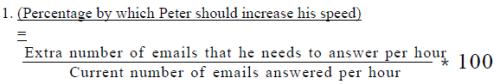2. So, to find the percentage by which Peter should increase his speed, we should first find the extra number of emails that he needs to answer.
3. For finding the extra number of emails that he needs to answer, we need to find the total number of emails currently answered by Peter, John and Mark together in 1 hour and number of increased emails they need to answer together.

• We are given the number of emails answered by Peter and Mark in 1 hour.
• As we know that John can answer 25 email in 5/6  hours, we can find the number of emails answered by John in 1 hour

Working out:

1. Number of emails answered by Mark in 1 hour = 25 + 40% of 25 = 25 + 10 = 35
2. Number of emails answered by John in 1 hour =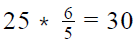3.  So, the number of emails currently being answered by Peter, mark and John combined = 25 + 35 + 30 = 90
4. Number of emails they should answer in case of 1/6 increase = 90 +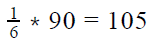emails
5. As there are 105 – 90 = 15 more emails that need to answered, these should be answered by Peter.
6. So, Peter should answer 15 more emails per hour
• Hence, Peter’s speed should increase by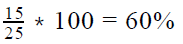So, Peter should increase his speed by 60%

QUESTION: 4

If John produces n units of an item in t hours, what is the value of n?

(1) If John produces 4 more items per hour, he would take 1/4 lesser time to produce n units of the item.
(2) If John increases his rate of production by 25 percent, the time taken by him to produce n items would be 80 percent of the original time.

Solution:

Step 1 & 2: Understand Question and Draw Inference

• Work done = Number of items produced by John = n
• Time taken = Time taken to produce n items = t hours
• Therefore, Rate of work done = Number of items produced in 1 hour = n/t

To Find: Value of n

Step 3 : Analyze Statement 1 independent

(1) If John produces 4 more items per hour, he would take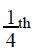lesser time to produce n units of the item.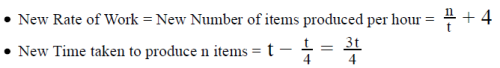• Number of items produced = n
• (Number of items produced) = (New Rate)*(New Time)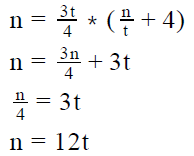However, we do not know the value of t, hence we cannot find the value of n.

Step 4 : Analyze Statement 2 independent

(2) If John increases his rate of production by 25 percent, the time taken by him to produce n items would be 80 percent of the original time.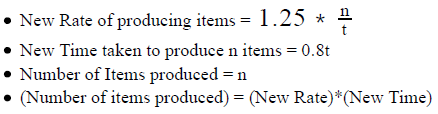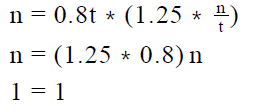Thus, there is no new information given. Insufficient to answer

Step 5: Analyze Both Statements Together (if needed)

1. n = 12t
2. No new information given

As statement-2 does not provide us any new information, the combination of the statements is insufficient to answer the question.

QUESTION: 5

Transmitting data at the same constant rate, 12 identical cables can transmit x bits of digital information per minute. How many bits of information can be transmitted by 27 such cables in 25 seconds?

Solution:

Given:

• Rate of transmission of 12 identical cables = x bits per minute
• Rate of transmission is constant

To Find: Number of bits (in terms of x) transmitted by 27 identical cables in 25 seconds

Approach:

1. Required number of bits = 27*(Number of bits transmitted by 1 cable in 25 seconds)
• = 27*(Number of bits transmitted by 1 cable in 1 second) *25
2. So, to answer the question, we need to find the number of bits transmitted by 1 cable in 1 second

Working out:

• Finding the number of bits transmitted by 1 cable in 1 second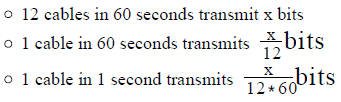• Finding the Required value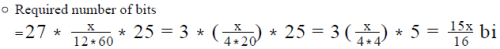Looking at the answer choices, we see that the correct answer is Option A

QUESTION: 6

The water from outlet A, flowing at a constant rate, can fill a rectangular swimming pool in 12 hours while the water from outlet B, flowing at a constant rate, can fill the same swimming pool in 18 hours. On a day when the pool was empty, outlet A was opened at 7 AM and outlet B was opened at 10 AM. Outlet A was closed at 2 PM and outlet B was closed at 4 PM. If there was no water flow from outlet A between 10:30 AM to 11:30 AM because of maintenance work, the height of the water in the pool at 4 PM was approximately what percentage of the depth of the pool?

Solution:

Given:

• Outlet A can fill a swimming pool in 12 hours
• Outlet B can fill the same swimming pool in 18 hours
• Outlet A was opened at 7 AM
• Outlet A was closed at 2 PM
• No water flow between 10:30 AM -11:30 AM, i.e. 1 hour
• Outlet B was opened at 10 AM
• Outlet B was closed at 4 PM

To Find: Percentage of swimming pool that is filled?

Approach: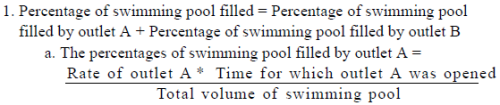b. As outlet A working alone can fill the entire swimming pool in 12 hours, we can write the volume of swimming pool = Rate of outlet
A * 11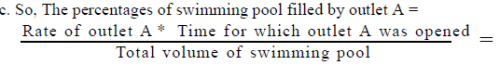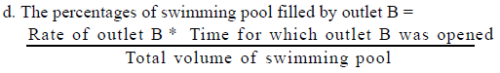e. As outlet B working alone can fill the entire swimming pool in 18 hours, we can write the volume of swimming pool = Rate of outlet
A * 18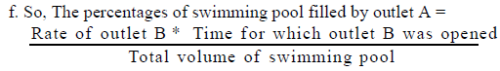2. As, we are given the time for which outlets A and B were open, we can find the percentage of swimming pool filled by outlet A and B

Working out:

1. Outlet A

a. Time at which outlet A was opened = 7 AM
b. Time for which outlet A was closed = 2 PM
c. However, outlet did not work for 1 hour between 10:30 AM - 11:30 AM
d. Hence outlet A worked for 7 – 1 = 6 hours
e. So, percentage of swimming pool filled by outlet A in 6 hours = 6/12 = 50% of the swimming pool

2. Outlet B
a. Time at which outlet B was opened = 10 AM
b. Time for which outlet B was closed = 4 PM
c. Time for which outlet B worked = 4 PM – 10 AM = 6 hours
d. So, percentage of swimming pool filled by outlet B in 6 hours = 6/18 = 33% of the swimming pool

3. Therefore, outlet A and B working for 6 hours each can fill (50% +33%) = 83% of the entire swimming pool

QUESTION: 7

If 30 machines, each working at the same constant rate, together can produce n/2  units of an item in 15 days, how many machines will be needed to produce 2n units of the item in 45 days?

Solution:

Given:

• Let the production rate of one machine = r units per day
• Number of machines working = 30
• Number of items produced by 30 machines = n/2 units
• Time taken by 30 machines to produce n/2 items = 15 days

To Find: Number of machines needed to produce 2n units of the item in 45 days

Approach:

1. Let the number of machines needed be x
2. Production rate of one machine = r units per day
• Production rate of x machines = rx units per day
3. We know that Work = Rate * Time
4. So, 2n = r * 45 * x
• ​​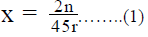• For finding the value of x, we need to either find the values of n and r, or the ratio n/r
5. As we are given the time taken by 30 machines to produce n/2 items, we can use this information to find the value of n/r.

Working out: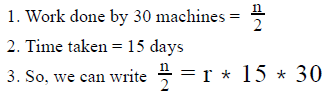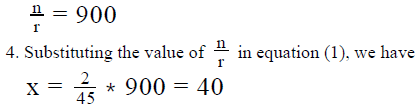5. So, it will take 40 machines to produce 2n units of the item in 45 days

QUESTION: 8

A rectangular tank has the dimensions of its base as 30 metres by 20 metres and a height of 10 metres. There are two taps attached to the tank such that each tap working alone at a constant rate can fill the tank completely in 60 hours and 90 hours respectively. One of the walls of the tank has holes along the height of the tank at a regular distance of 2 metres and the first such hole is 2 metres above the base of the tank. The rate of water outflow from each hole is 10m per hour. If both the taps are opened simultaneously in the empty tank, approximately how many hours will it take to fill the tank completely?

Solution:

Given:

• Length of rectangular tank = 30 meters
• Breadth of rectangular tank = 20 meters
• Height of rectangular tank = 10 meters
• Time taken by tap-1 to fill the entire tank = 60 hours
• Time taken by tap-2 to fill the entire tank = 90 hours
• Holes are at a height of 2 meters
• Height of first hole from the base = 2 meters
• Height of 2nd hole from the base = 4 meters
• Height of 3rd hole from the base = 6 meters
• Height of 4th hole from the base = 8 meters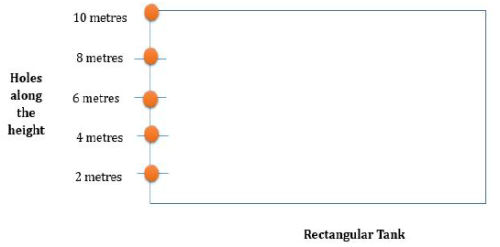• Rate of water outflow from each hole = 10m3 per hour

To Find: Time taken to fill the empty tank completely if both the taps are opened?

Approach:

1. Work done = Rate * Time taken
• We know that work done is to fill the entire tank
• So, for finding the time taken, we need to find the rate of doing work.
2. Rate of doing work = Rate of inflow by both the taps – Rate of outflow by the holes
3. Rate of Inflow
1. As we are given the time taken by both the taps individually to fill the tank, we can find the combined rate of ttaps to fill the tank.
• Combined rate of the taps = Rate of tap-I + Rate of tap-II
4. Rate of Outflow
• However, the rate of outflow will not be constant throughout the tank, as it will depend on the number of holes from which the water is flowing out.
• For example, increasing the water level in tank from 2 meters to 4 meters will have a different rate than increasing the water level from 4 metres to 6 meters. This is because the number of holes from which the water is outflowing is different in both the cases.
• So, we will need to find the time taken separately to fill the tank between each of the holes
5. Once we know the rate of inflow and the rate of outflow between each consecutive hole, we can find the net rate = Rate of inflow – Rate of outflow.
6. Using the net rate, we can find the time taken to fill the tank between each consecutive hole.
7. So, the total time taken to fill the tank = Sum of time taken to fill the tank between each consecutive hole.

Working out:

1. Total work to do done = Total volume to be filled = Volume of tank = 30 *20 *10 = 6000 m3
2. Rate of Inflow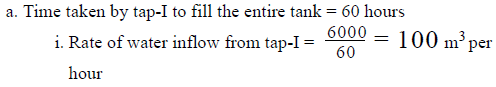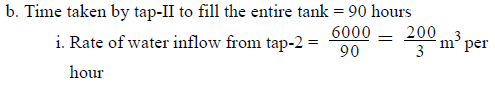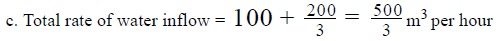3. Time Taken :

•  As the holes are at an interval of 2 meters, we will calculate the time taken for 2 meters at a time.
• Hence the volume of water needed for increasing the height of the water by 2 meters in the tank  30 *20*2 m3
•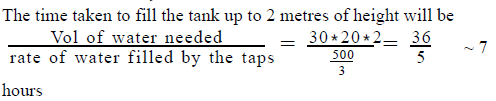•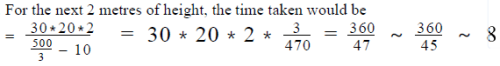• We have subtracted 10 in the net rate because from 1 hole there would be outflow of water at a rate of 10 m3 per hour
•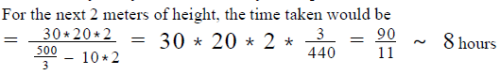• We have subtracted 10*2 in the net rate because from 2 holes there would be outflow of water each at a rate of 10 m3 per hour
•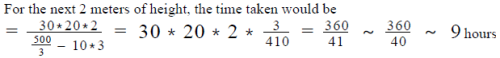• We have subtracted 10*3 in the net rate because from 3 holes there would be outflow of water each at a rate of 10 m3 per hour
•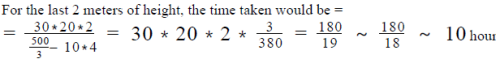• We have subtracted 10*4 in the net rate because from 4 holes there would be outflow of water each at a rate of 10 m3 per hour
4. Hence the total time taken would be ~ 7 +8+8+9+10 = 42 hours.

QUESTION: 9

4 identical machines, which operate at the same constant rate, together started manufacturing the batch of an item at 2 PM. Did
they finish the batch before 3.30 PM?
(1) The batch size was between 200 and 210 units, inclusive, of the item
(2) One machine could manufacture between 30 and 50 units, inclusive, of the item in 1 hour

Solution:

Step 1 & 2: Understand Question and Draw Inference

Given: Number of machines = 4
Let the rate of production for each machine = R units per hour

• So, the number of units produced by 4 machines in 1 hour = 4R

Let the batch size be B units

• Time taken by 4 machines to make 4R units = 1 hour
• So, time taken by 4 machines to make 1 unit = 1 4R  hours
• So, the time taken by 4 machines to produce B units =B4R hours

To Find: Did the machines finish the batch before 3:30 PM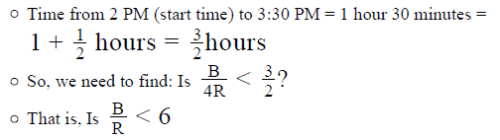Step 3 : Analyze Statement 1 independent

Statement 1 says that ‘The batch size was between 200 and 210 units, inclusive
of the item’

• So, 200 ≤ B ≤ 210
• However, since we do not know the value of R yet, we cannot answer the question
• Statement 1 is not sufficient

Step 4 : Analyze Statement 2 independent

Statement 2 says that ‘One machine could manufacture between 30 and 50 units, inclusive, of the item in 1 hour’

• So, 30 ≤ R ≤ 50
• However, since we do not know the value of B yet, we cannot answer the question
• Statement 2 is not sufficient

Step 5: Analyze Both Statements Together (if needed)

• From Statement 1: 200 ≤ B ≤ 210
• From Statement 2: 30 ≤ R ≤ 50
• Therefore, the minimum possible value of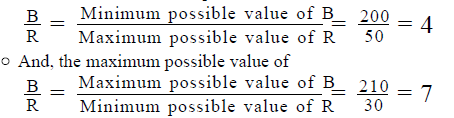• Thus, by combining the 2 statements, we see that the value of B/R lies between 4 and 7, inclusive
• So, the answer to the question ‘Is Is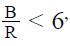may be YES or NO, depending on the particular values of B and R
• So, the 2 statements together are not sufficient to answer the question

QUESTION: 10

A container is completely filled with a sugar solution composed of water and sugar syrup in the ratio of 7:3. The container has 2 holes covered with filters such that from one of the holes only sugar syrup can flow out and from the other hole only water can flow out. The rates of water outflow and sugar syrup outflow from their respective holes are x cubic centimeters per hour and y cubic centimeters per half an hour respectively, such that x:y = 5:1. If the water from the solution can be drained out completely in 14 hours from the hole, how much time in hours would it take the container to be empty if both the holes are opened simultaneously?

Solution:

Given:

• Let the volume of the container be S liters
• Since the container is completely filled with the sugar solution, the volume of sugar solution will also be S liters
• Volume of water = 0.7S
• Volume of sugar syrup = 0.3S
• Rate of water outflow = x cubic centimeters per hour
• Water can be drained out completely in 14 hours
• Rate of sugar syrup outflow = y cubic centimeters per half an hour
• Rate of sugar syrup outflow = 2y cubic centimeters per hour x: y = 5:1

To Find: Time taken for container to be empty, if both the holes are opened simultaneously?

Approach:

1. As both the holes are opened simultaneously, the time taken to empty the container = Max( Time taken for sugar syrup to drain completely, Time taken for water to drain completely)
2. So, we need to find the individual time taken for both water and sugar syrup to drain out completely
• As we are given the time taken for water to drain out completely, we need to find the time taken for sugar syrup to drain out completely
3. Time taken for sugar syrup to drain completely =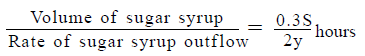• So, we need to find either the values of S and y or the ratio S/Y
4. Now, we are given that x : y = 5: 1, so if we can find the ratio of S/X, we can find the ratio of S/Y
5. Also, since we know that water can be drained out completely in 14 hours, we can calculate the ratio of S/X by using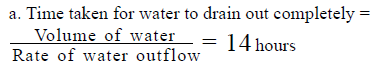Working out: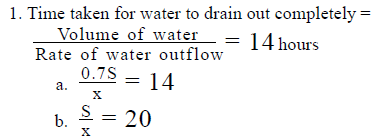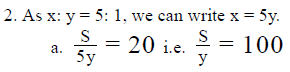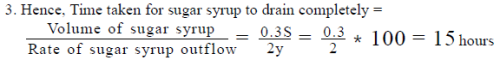4. So, let’s compare the time taken for water and sugar syrup to drain out completely
a. Time taken for water to drain out completely = 14 hours
b. Time taken for sugar syrup to drain out completely = 15 hours
5. Hence, the time taken for the container to be empty = Max( 14, 15) = 15 hours
Therefore, the container would be completely empty in 15 hours

QUESTION: 11

An automated manufacturing plant uses robots to manufacture products. A generation-I robot working alone can manufacture a product in 30 hours whereas a generation-II robot working alone can manufacture the same product in 20 hours. If the manager of the plant wants the product manufacturing time to be greater than equal to 5 hours with at least 1 robot each of both generations working together, how many possible combinations of the number of robots of each generation is possible?

Solution:

Given:

• Generation-I Robot
• Time taken to manufacture a product = 30 hours
• Generation-II Robot
• Time taken to manufacture the product = 20 hours
• To Find: Possible combinations of number of generation-I and II robots such that the product manufacturing time ≥ 5 hours
• Let the number of generation-I robots be x and number of generation-II robots be y

Approach:

1. We need to find the possible combination of the values of (x, y).
2. As we are given that the time taken for x generation-I and y generation-II robots should be greater than equal to 5 hours, we will formula an inequality in time taken for x generation robots and y generation-II robots to evaluate the possible combination of (x, y)
3. As Work = Rate * Time, for finding the time taken by the robots, we would need to find the work done and the rate
4. Number of products to be produced = 1
5. Now, we know that rate of production of n robots = n * rate of production of 1 robot.
6. So, once we find the rate of production of 1 robot for each generation, we can find the rate of production of x generation-I robots and y generation-II robots respectively.
7. As we are given the time taken by 1 generation-I robot and 1 generation-II robot to produce 1 product each, we can find the rate of production for robots of both generations.

Working out:

Generation-I
a. Work Done = 1 product
b. Time taken = 30 hours
c. Rate of manufacturing products = products per hour

Generation-II
a. Work Done = 1 product
b. Time taken = 20 hours
c. Rate of manufacturing products = 1/20 products per hour
3. Let’s assume there are x generation-I robots and y generation-II robots working together to produce 1 unit of product
a. So, work done = 1 product

b. Rate of x generation-I robots  =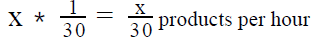c. Rate of y generation-II robots =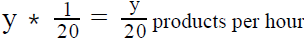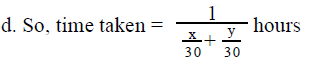4. As the time taken ≥ 5 hours, we can write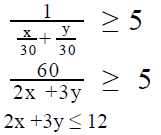5. So, the possible combinations of {x, y} can be:
a. If y = 1, x = {1,2,3,4} – 4 options
b. If y = 2, x = {1,2,3} – 3 options
c. If x = 1, y = {1,2,3} – 3 options
d. However {x, y} = {1,1} and {1,2} is repeated.

6. So, we have a total of 10 -2 = 8 possible options
a. Please note that we have not considered x, y = 0 as we are given that at-least 1 robot of each generation should be working

QUESTION: 12

Two machines A and B worked at variable rates. The average number of items produced per day by machine A could be expressed as Ra (x) = ax(x + 1) and the average number of items produced per day by machine B could be expressed as Rb (x) = bx(x-1), where x was the number of consecutive days worked and a, b were constants. If machine B produced 90 more items when it worked for 4 consecutive days than when it worked for to 3 consecutive days and machine A produced a total of 232 items when it worked in two intervals of 3 consecutive days and 4 consecutive days, how many items was produced by machines A and B working together for 10 consecutive days?

Solution:

Given:

• Average number of items produced per day by machine A , Ra (x) = ax(x + 1)
• x is the number of consecutive days worked and a is a constant
• Machine A produced 232 items when it worked for two intervals of 3 consecutive days and 4 consecutive days
• Average number of items produced per day by machine B, Rb (x) = bx(x-1)
• x is the number of consecutive days worked and b is a constant
• Machine B produced 90 more items when it worked for 4 consecutive days than when it worked for 3 consecutive days

To Find: Number of items produced by machine A and B working together for 10 consecutive days

Approach:

1. We know that Number of items produced = Rate of producing items * Time taken
• As we are given the time taken to be 10 days, we need to find the rate of producing items for both machines A and B, i.e. we need to find the values of Ra (x) and Rb (x)
2. For finding the values of R (x) and R (x), we need to find the values of a and b, i.e. we need an equation each in a and b.
3. As we are given the number of items produced by machine A in a certain time interval and the number of items produced by machine B in a certain time interval, we will use this information to find the values of a and b.

Working out:

1. Rate of producing items of machine A
• Number of items produced working for 3 consecutive days = ax(x+1)*x = 3a(3+1) * 3 = 36a
• Number of items produced working for 4 consecutive days = ax(x+1)*x= 4a(4+1) * 4 = 80a
•  So, total number of items produced = 36a +80a = 116a = 232
• a = 2
2. Rate of producing items of machine B
• Number of items produced working for 4 consecutive days = bx(x-1)*x = 4a(4-1) * 4 = 48b
• Number of items produced working for 3 consecutive days = bx(x-1)*x = 3a(3-1) * 3 = 18b
• So, extra items produced = 48b – 18b = 30b = 90
• b = 3
3. Number of items produced by machine A working for 10 consecutive days = ax(x+1)*x = 2*10 *(10+1)*10 = 2200
4. Number of items produced by machine B working for 10 consecutive days = bx(x-1)*x = 3*10*(10-1)*10 = 2700
5. Total number of items produced by machine A and machine B working together for 10 consecutive days = 2200 + 2700 = 4900

QUESTION: 13

John starts reading a book from the first page onwards and reads each page only once. Every day, he reads twice the number of pages read by him the previous day. If the book has 400 pages, will John still be reading the book on the 6 day after he starts reading it?
(1) On the fifth day of reading the book, John reads more than 180 pages
(2) John reads 52 more pages on the fourth day of reading the book than he reads on the third day

Solution:

Step 1 & 2: Understand Question and Draw Inference

• Let’s assume John reads x pages on the first day.
• Number of pages read by John on second day = 2x
• Number of pages read by John on the third day = 22 x
• Number of pages read by John on 4th day = 23 x
• Number of pages read by John on 5th day = 24 x
• Total pages read by John in 5 days = x +2x +4x +8x +16x = 31x
• Number of pages in the book = 400

To Find: Is 31x ≥ 400 ?

• If Yes, then John will have finished the book before 6 day. So, he will not be reading the book on the 6 day
• If No, then John will still be reading the book on the 6 day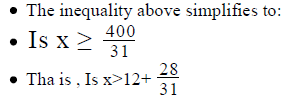Step 3 : Analyze Statement 1 independent

• On the fifth day of reading the book, John reads more than 180 pages
• Number of pages read on 5 day = 16 x> 180.
• x > 11
• So, x = 12 < 12. Something or x = 13 > 12.something

Step 4 : Analyze Statement 2 independent

(2) John reads 52 more pages on the fourth day of reading the book than he reads on the third day

• Number of pages read on third day = 4x
• Number of pages read on fourth day = 8x
• Extra number of pages read on the fourth day compared to the third day = 8x – 4x = 4x

4x = 52, i.e. x = 13

Therefore, the answer to the question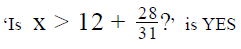Step 5: Analyze Both Statements Together (if needed)

As we have a unique answer from step-4, this step is not required.

QUESTION: 14

Two machines A and B work at constant rates which are in the ratio of 1:2 respectively. If the two machines work together, they can complete 1/3rd of a job in 3 hours. If machine A starts working on the job and works alone for 18 hours, how many hours would machine B working alone take to complete the remaining work?

Solution:

Given:

• Let the rate of machine A be x units of work per hour
• Therefore the rate of machine B = 2x units of ork per hour
• Let the total amount of work to be done be W units
• Time taken by machines A and B working together to complete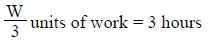• Machine A works alone for 18 hours

To Find: Time taken by machine B to complete the remaining work

Approach:

1. We know that Work = Rate * Time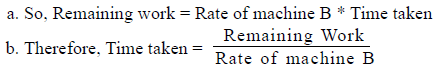c. So, to find the time taken, we either need to find the amount of remaining work and the rate of machine B or the ratio of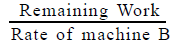2. For finding the remaining work, we need to find the amount of work done by machine A in 18 hours

• Remaining work = W – work done by machine A in 18 hours
• Work done by machine A in 18 hours = Rate of machine A * 18
• As, rate of machine A is in terms of x, work done by machine A in 18 hours can be expressed in terms of x
• Remaining work = W – work done by machine A in 18 hours
• This will be an expression in terms of W and x. As the rate of machine B is in terms of x, we need to express W also in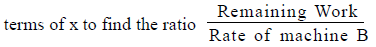3. We are given that machines A and B working together take 3 hours to  complete W/3  units of work.

•  We will use the above relation to express W in terms of x

Working out:

1. Machines A and B working together take 3 hours to complete W/3 units of work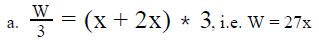2. Amount of work done by machine A working alone for 18 hours = x * 18 = 18x

3. Remaining work = W – 18x = 27x – 18x = 9x
4. Amount of time taken by machine B to complete the remaining work =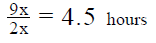QUESTION: 15

Machines M and N produce identical widgets but at different constant rates. Machine M takes 10 hours to produce 1000 widgets and Machines M and N together take 6 hours and 40 minutes to produce 2000 widgets. How much time does Machine N take to produce 3000 widgets?

Solution:

Given:

• Let rate of machine N be n widgets per hour and let the rate of Machine M be m widgets per hour
• m ≠ n
• Number of widgets produced by M in 10 hours = 1000
• Number of widgets produced by M and N together in 6 hours 40 minutes = 2000

To Find: Time taken by N to produce 3000 widgets

Approach: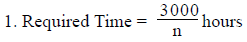• So, to answer the question, we need to know the value of n

2. We are given the Combined output of M and N in 6 hours 40 minutes. From this piece of information, we can find the combined Rate of M and N

a. Combined Rate of M and N = m + n
b. So, n = (Combined Rate of M and N) – m
c. Therefore, we need to find not only the Combined Rate of M and N, but also the value of m

3. We’re given the output of M alone in 10 hours. From this piece of information, we can find the value of m.

Working out:

• Finding the Combined Rate of M and N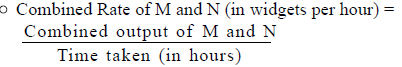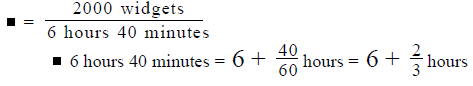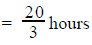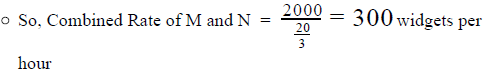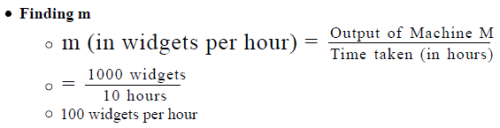• Finding n
• n = (300 – 100) widgets per hour = 200 widgets per hour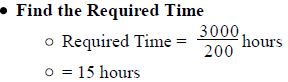Looking at the answer choices, we see that the correct answer is Option E

QUESTION: 16

A rectangular reservoir is filled with water till one-fifth of the height of the reservoir. If an outlet at the bottom of the reservoir is unplugged, the water in the reservoir will drain completely in 1 hour. If the outlet remains plugged and the inlet tap at the head of the reservoir is opened, the reservoir will fill to the brim in 2 hours. In how much time will the reservoir fill to the brim if the outlet is unplugged 30 minutes after the inlet tap is opened?

Solution:

Given: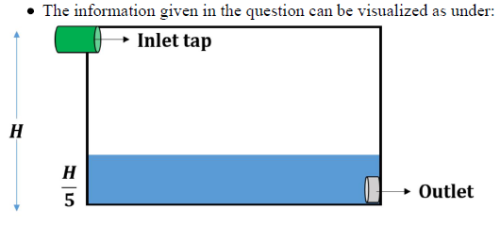• Let the length and the breadth (of the base) of the reservoir be L and B, and let its height be H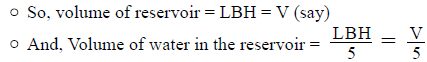• Time taken to drain the water in the reservoir is 1 hour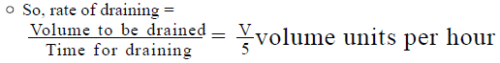• Time taken to fill the reservoir completely (when  V/5 water is already there) is 2 hours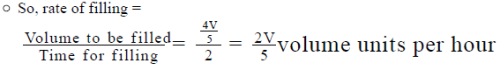To Find: Time taken to fill the reservoir if outlet is unplugged 30 minutes after inlet is opened

Approach: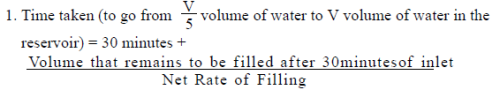• Here, 30 minutes is the time for which only the inlet pipe is opened
• (Volume that remains to be filled after 30 minutes of inlet) = (Volume that initially needed to be filled) – (Volume of water pumped in through the inlet in 30 minutes)
• And, Net Rate of Filling = Rate of Filling – Rate of Draining

Working out:

• Finding the Volume that Remains to be filled after 30 minutes of inlet
• (Volume that remains to be filled after 30 minutes of inlet) = (Volume that initially needed to be filled) – (Volume of water pumped in through the inlet in 30 minutes)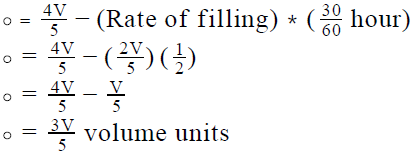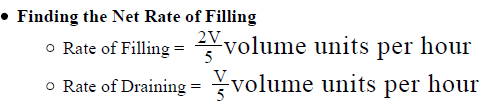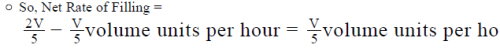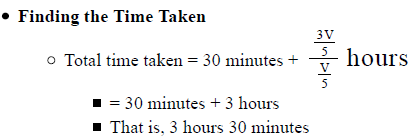Looking at the answer choices, we see that the correct answer is Option B

QUESTION: 17

On the first day of the launch of an anticipated electronic item, a queue formed outside an exclusive electronics store that sold the item. At the time the store opened, the queue had 60 people in it and throughout the day, a new person joined the queue every 3 minutes. That day, the store served, only the people in the queue, at a constant service rate of 30 people per hour. If no person re-joined the queue upon getting served once, how many people were in the queue 4 hours after the store opened?

Solution:

Given:

• Number of people in the queue when the store opened = 60
• 1 person joins the queue every 3 minutes
• So, Queue Joining Rate = 1 person per 3 minutes
• Constant service rate = 30 people per hour
• So, 30 people exit the queue each hour

To Find: Number of people in the queue 4 hours after the store opened

Approach:

1. Number of people in the queue 4 hours after the store opened = (Number of people in the queue when the store opened) + (Number of people who join the queue in 4 hours) – (Number of people who exit the queue in 4 hours)
• Number of people who join the queue in 4 hours = (Queue Joining Rate, in terms of people per hour)* 4
• (Number of people who exit the queue in 4 hours) = (Service Rate, in terms of people per hour)*4
2. We’re already given the number of people in the queue when the store opened and the Service Rate, in terms of people per hour. So, the only piece of information that we need to find is the Queue Joining Rate, in terms of people per hour

Working out:

• Finding Queue Joining Rate, in terms of people per hour
• Number of people who join in 3 minutes = 1
• Number of people who join in 60 minutes  =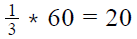• So, the Queue Joining Rate = 20 persons per hour
• Finding the Required Value

• Number of people in the queue 4 hours after the store opened = (Number of people in the queue when the store opened) + (Number of people who join the queue in 4 hours) – (Number of people who exit the queue in 4 hours)
• Number of people in the queue 4 hours after the store opened = 60 + 20*4 – 30*4
• = 60 – 10*4
• =60 – 40
• = 20

Looking at the answer choices, we see that the correct answer is Option A

QUESTION: 18

A is thrice as good as B in work. A is able to finish a job in 60 days less than B. They can finish the work in - days if they work together.A.

Solution:

If A completes a work in 1 day, B completes the same work in 3 days
Hence, if the difference is 2 days, B can complete the work in 3 days
=> if the difference is 60 days, B can complete the work in 90 days
=> Amount of work B can do in 1 day= 1/90
Amount of work A can do in 1 day = 3 × (1/90) = 1/30
Amount of work A and B can together do in 1 day = 1/90 + 1/30 = 4/90 = 2/45
=> A and B together can do the work in 45/2 days = 22 ½ days

QUESTION: 19

A can do a particular work in 6 days . B can do the same work in 8 days. A and B signed to do it for Rs. 3200. They completed the work in 3 days with the help of C. How much is to be paid to C?A.

Solution:

Amount of work A can do in 1 day = 1/6
Amount of work B can do in 1 day = 1/8
Amount of work A + B can do in 1 day = 1/6 + 1/8 = 7/24
Amount of work A + B + C can do = 1/3
Amount of work C can do in 1 day = 1/3 - 7/24 = 1/24
work A can do in 1 day: work B can do in 1 day: work C can do in 1 day
= 1/6 : 1/8 : 1/24 = 4 : 3 : 1
Amount to be paid to C = 3200 × (1/8) = 400

QUESTION: 20

6 men and 8 women can complete a work in 10 days. 26 men and 48 women can finish the same work in 2 days. 15 men and 20 women can do the same work in - days.

Solution:

Let work done by 1 man in 1 day = m and work done by 1 woman in 1 day = b
Work done by 6 men and 8 women in 1 day = 1/10
=> 6m + 8b = 1/10
=> 60m + 80b = 1 --- (1)
Work done by 26 men and 48 women in 1 day = 1/2
=> 26m + 48b = ½
=> 52m + 96b = 1--- (2)
Solving equation 1 and equation 2. We get m = 1/100 and b = 1/200
Work done by 15 men and 20 women in 1 day
= 15/100 + 20/200 =1/4
=> Time taken by 15 men and 20 women in doing the work = 4 days